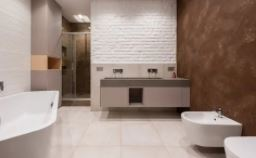#### How to Calculate the Number of Floor Tiles You Need for Your Adelaide HomeWhen we talk about calculating the number of floor tiles, it doesn’t necessarily mean you’ll get an accurate number. The calculations to know the number of floor tiles you’ll need for your Adelaide home help you to see if you’ll need a lot of tiles or not. The calculations will just be giving you an idea of how many tiles you’ll need.

Once you have a general idea of how many tiles you’ll be needing, you’ll be able to adjust your budget to fit your home’s needs.

Before you start your calculations, you’ll need some materials that you’ll be using for your estimate;

• Paper
• Pen or pencil, but pencil is advisable to make corrections easily if you make a mistake.
• Measuring tape
• Calculator

Some things you’ll need to know to calculate the number of tiles you’ll be needing in your Adelaide home are:

## Correct Measurements

To make calculations, you’ll need figures. You’ll get the figures you need in calculating the number of floor tiles you’ll need in your Adelaide home by taking measurements. Your measurements determine the accuracy of your calculations.

What units of measurement are you using? First, you’ll need to find out the square footage of all the rooms in your Adelaide home, or at least all the rooms you want to install tiles in.

Calculating the square footage of a room is easy; multiply the room’s length by the width of the room. If the size of the room is 20 and the breadth is 16. It’ll be 20 16 = 320 square feet.

It’s advisable to do your measurements in feet, so the calculation is more effortless. However, when you measure in inches, you’ll divide whatever measurements you get by 12 before you finally multiply to get your square footage.

Here are different types of measurement you’ll be taking:

• Vertical measurements: You’ll measure the wall from top to bottom for wall projects in the kitchen or bathroom. Start your measurement from where you want the wall tiles to start. The same thing applies to floor tiles; measure the room’s length you want the floor tiles to be installed in.
• Horizontal measurements: This measurement is from left to right and spans the breadth of the room you want them in. It applies to wall and floor tiles.

These measurements are what you’ll be using to get all your calculations; try to make them as accurate as possible.

## The Number Of Tiles You’ll Need

Tiles come in boxes, and you’ll be needing multiple tile boxes for your floor tiles. Let’s say each tile box contains 12 square feet of tile. Using our former measurement of the 320 square feet room, you’ll divide the square foot of the room by the square feet of the tile boxes. So for this room, you’ll be needing at least 26 boxes.

Basic knowledge about calculating the number of floor tiles is okay; whoever you want to buy the tiles from will give you the accurate measurement. For example, when you buy from Aurees tiles, we’ll provide you transparent figures on the number of tiles you’ll need; we’re more focused on satisfying our customers than making a profit.